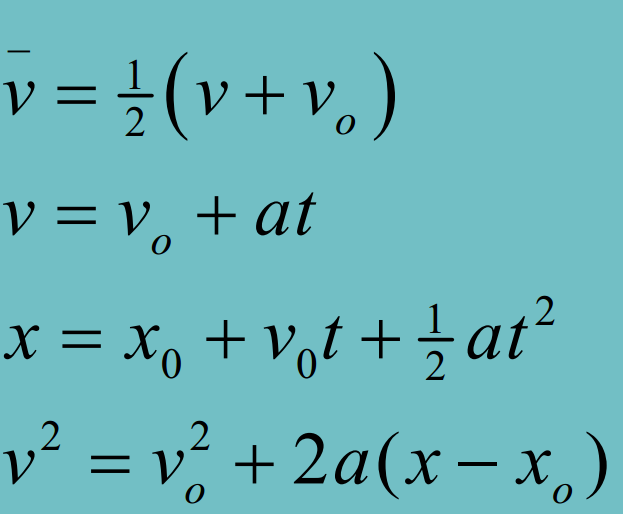# MIDTERM NOTES

Created Wednesday 25 May 2016Dimension: Classification/Nature of physical quantity
Unit: Standard of measurement of a physical quantity

Dimensions
distance -> L
time -> T
mass -> M

Dimensional homogeneity means only quantities with the same dimensions may be compared, equated, added, or subtracted.
Basically only stuff with same dimensions can be used mathematically.

Quantity SI Unit Other SI Unit Dimension
Force kgms^-2 N MLT^-2
Energy kgm^2s^-2 J ML^2T^-2
Power kgm^2s^-3 W ML^2T^-3
Momentum kgms^-1   MLT^-1
Impulse kgms^-1   MLT^-1

----------

## MIDTERM PREP STARTS HERE

----------

#### PDF 1

Distance != Displacement

Average Speed != Average velocity

Speed -> Distance
Velocity -> Displacement

Average acceleration -> Change in velocity

Negative acceleration != Deceleration

"Negative acceleration is acceleration in the negative direction
as defined by the coordinate system."

"Deceleration occurs when the acceleration is opposite in
direction to the velocity (i.e. slowing down)"

Integration gets you the area under the curve

for relative velocity

V(BW) is the velocity of the boat in the water frame - it is equal to V(B)-V(W)